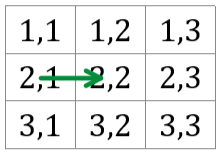# Akash and Grid solution codechef

## Akash and Grid solution codechef

Akash is stuck in a N×NN×N grid, where NN is odd. The rows of the grid are numbered 11 to NN from top to bottom, and the columns are numbered 11 to NN from left to right. The cell at the intersection of the ii-th row and jj-th column will be denoted (i,j)(i,j).

The grid has a unique center cell — ((N+1)/2,(N+1)/2)((N+1)/2,(N+1)/2). For example, when N=5N=5 the center is cell (3,3)(3,3).

Akash is currently at cell (xs,ys)(xs,ys). He would like to reach the exit of the grid, which is located at the center. It is guaranteed that (xs,ys)(xs,ys) is not the center.

Suppose Akash is at cell (x,y)(x,y). He can make the following movements:

• He can freely move along diagonals, i.e, to cells (x1,y1),(x1,y+1),(x+1,y1),(x+1,y+1)(x−1,y−1),(x−1,y+1),(x+1,y−1),(x+1,y+1)
• He can move one step horizontally or vertically with the cost of 11 gold coin, i.e, to cells (x,y1),(x,y+1),(x1,y),(x+1,y)(x,y−1),(x,y+1),(x−1,y),(x+1,y)

Note that Akash is not allowed to make a move that will take him out of bounds of the grid.

Akash would like to minimize the number of coins required to reach the center. Please help him find this number.

### Input Format

• The first line of input contains a single integer TT, denoting the number of test cases. The description of TT test cases follows.
• Each test case consists of a single line of input, containing three space-separated integers N,xs,ysN,xs,ys — the size of the grid and the coordinates of Akash’s starting cell.

### Output Format

For each test case, output in a single line the minimum number of gold coins Akash needs to reach the center.

### Constraints

• 1T1041≤T≤104
• 3N<21043≤N<2⋅104
• NN is always odd.
• 1xs,ysN1≤xs,ys≤N

### Sample Input 1

2
3 2 1
5 3 1


### Sample Output 1

1
0


### Explanation

Test case 1: For a 3×33×3 grid, the center is at (2,2)(2,2). It is not possible to reach (2,2)(2,2) from (2,1)(2,1) using only diagonal moves. So, Akash will directly go to the center using 1 gold coin.Test case 2: N=5N=5, so the center is (3,3)(3,3). Akash can go from (3,1)(3,1) to (2,2)(2,2) with a diagonal move, then from (2,2)(2,2) to (3,3)(3,3) with another diagonal move. So, he needs zero coins.